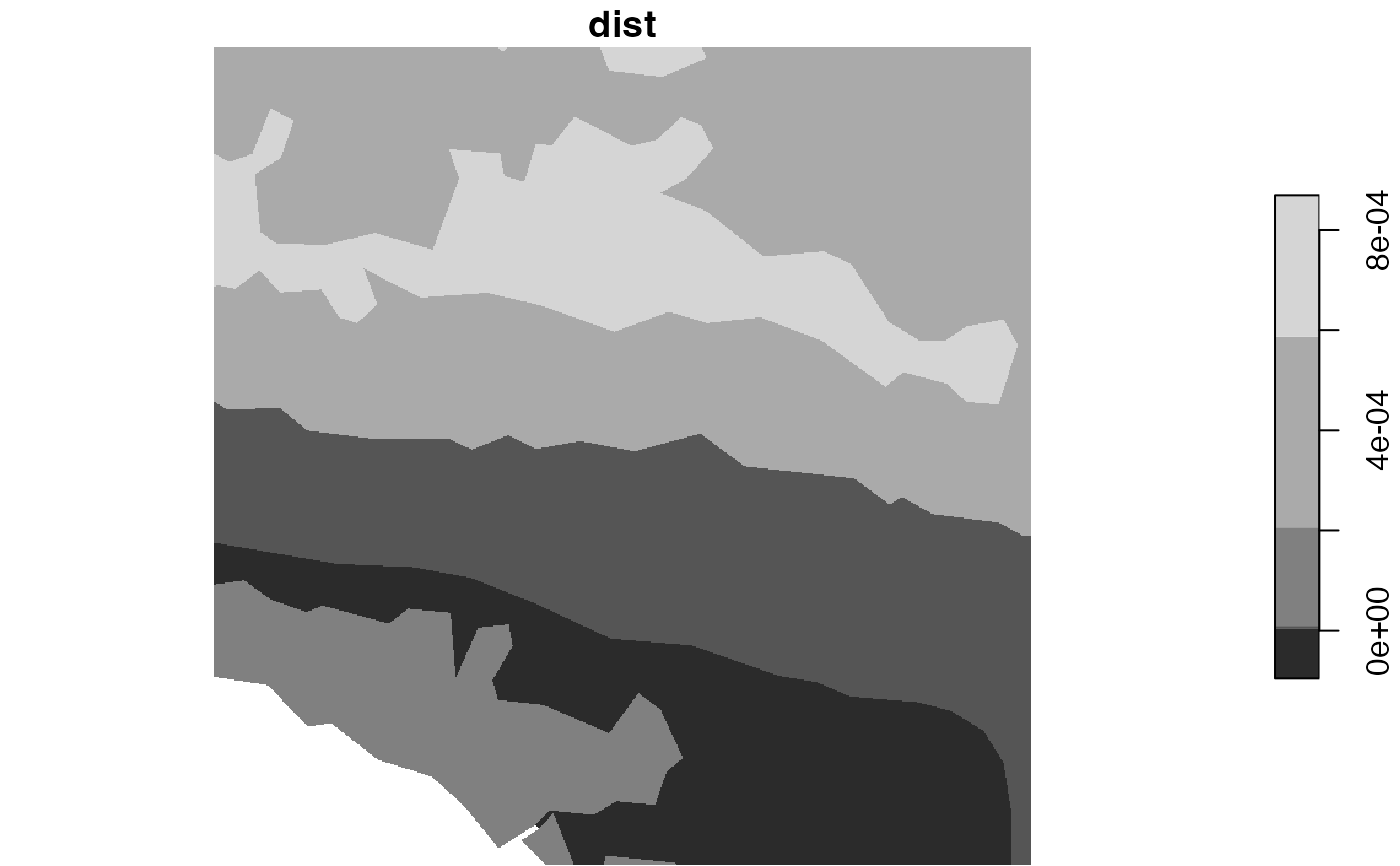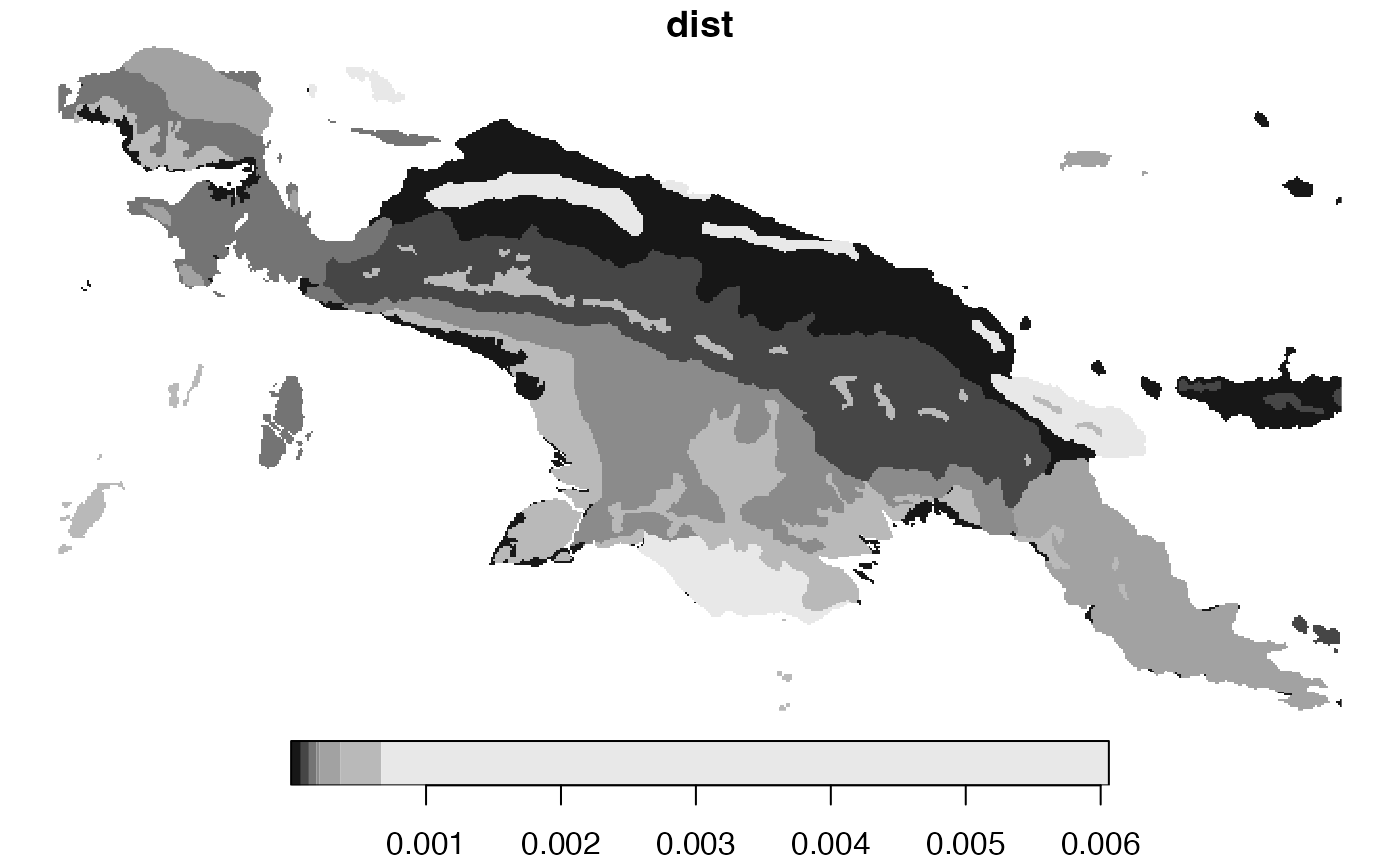Compares two spatial datasets containing categorical raster data. It accepts a categorical raster dataset with one or more attributes, and compares it to the second dataset with the same attributes and dimensions. The both dataset are either compared to as whole areas, areas divided into regular windows, or areas divided into irregular windows. This function allows for several types of comparisons using different representations of spatial patterns, including "coma" (co-occurrence matrix), "cove" (co-occurrence vector), "cocoma" (co-located co-occurrence matrix), "cocove" (co-located co-occurrence vector), "wecoma" (weighted co-occurrence matrix), "wecove" (weighted co-occurrence vector), "incoma" (integrated co-occurrence matrix), "incove" (integrated co-occurrence vector). These representations are created for both datasets, and next a distance between them is calculated using a selected measure from the philentropy::distance function. Additional parameters, such as neighbourhood or normalization types, are also available.

lsp_compare(
x,
y,
type,
dist_fun,
window = NULL,
output = "stars",
neighbourhood = 4,
threshold = 0.5,
ordered = FALSE,
repeated = FALSE,
normalization = "pdf",
wecoma_fun = "mean",
wecoma_na_action = "replace",
...
)

## Arguments

x

Object of class stars, stars_proxy, or terra's SpatRaster. It should have one attribute (for "coma", "cove"), two attributes ("cocoma", "cocove", "wecoma", "wecove"), two or more attributes ("incoma", "incove"), or any number of attributes suitable for user-defined functions.

y

Object of class stars, stars_proxy, or terra's SpatRaster. It should have one attribute (for "coma", "cove"), two attributes ("cocoma", "cocove", "wecoma", "wecove"), two or more attributes ("incoma", "incove"), or any number of attributes suitable for user-defined functions.

type

Type of the calculated signature. It can be "coma" (co-occurrence matrix), "cove" (co-occurrence vector), "cocoma" (co-located co-occurrence matrix), "cocove" (co-located co-occurrence vector), "wecoma" (weighted co-occurrence matrix), "wecove" (weighted co-occurrence vector), "incoma" (integrated co-occurrence matrix), "incove" (integrated co-occurrence vector), "composition" or any function that can summarize stars objects.

dist_fun

Distance measure used. This function uses the philentropy::distance function in the background. Run philentropy::getDistMethods() to find possible distance measures.

window

Specifies areas for analysis. It can be either: NULL, a numeric value, or an sf object. If window=NULL calculations are performed for a whole area. If the window argument is numeric, it is a length of the side of a square-shaped block of cells. Expressed in the numbers of cells, it defines the extent of a local pattern. If an sf object is provided, each feature (row) defines the extent of a local pattern. The sf object should have one attribute (otherwise, the first attribute is used as an id).

output

The class of the output. Either "stars", "sf", or "terra"

neighbourhood

The number of directions in which cell adjacencies are considered as neighbours: 4 (rook's case) or 8 (queen's case). The default is 4.

threshold

The share of NA cells to allow metrics calculation.

ordered

For "cove", "cocove", "wecove" and "incove" only. The type of pairs considered. Either "ordered" (TRUE) or "unordered" (FALSE). The default is FALSE.

repeated

For "incove" only. Should the repeated co-located co-occurrence matrices be used? Either "ordered" (TRUE) or "unordered" (FALSE). The default is FALSE.

normalization

For "cove", "cocove", "wecove", "incove", "composition", or user-provided functions only. Should the output vector be normalized? Either "none" or "pdf". The "pdf" option normalizes a vector to sum to one. The default is "pdf".

wecoma_fun

For "wecoma" and "wecove" only. Function to calculate values from adjacent cells to contribute to exposure matrix, "mean" - calculate average values of local population densities from adjacent cells, "geometric_mean" - calculate geometric mean values of local population densities from adjacent cells, or "focal" assign a value from the focal cell

wecoma_na_action

For "wecoma" and "wecove" only. Decides on how to behave in the presence of missing values in w. Possible options are "replace", "omit", "keep". The default, "replace", replaces missing values with 0, "omit" does not use cells with missing values, and "keep" keeps missing values.

...

Additional arguments for the philentropy::distance function.

## Value

Object of class stars (or sf or terra's SpatRaster, depending on the output argument). It has four attributes: (1) id - an id of each window. For irregular windows, it is the values provided in the window argument, (2) na_prop_x - share (0-1) of NA cells for each window in the x object, (3) na_prop_y - share (0-1) of NA cells for each window in the y object, (4) dist- calculated distance between signatures for each window

## Examples

library(stars)

lc15 = read_stars(system.file("raster/landcover2015s.tif", package = "motif"))
lc01 = read_stars(system.file("raster/landcover2001s.tif", package = "motif"))
ecoregions = read_sf(system.file("vector/ecoregionss.gpkg", package = "motif"))

ecoregions = st_transform(ecoregions, st_crs(lc15))

c1 = lsp_compare(lc01, lc15, type = "cove",
dist_fun = "jensen-shannon", window = ecoregions["id"])
plot(c1["dist"])# \donttest{
# larger data example
library(stars)

lc15 = read_stars(system.file("raster/landcover2015.tif", package = "motif"))
lc01 = read_stars(system.file("raster/landcover2001.tif", package = "motif"))
ecoregions = read_sf(system.file("vector/ecoregions.gpkg", package = "motif"))

ecoregions = st_transform(ecoregions, st_crs(lc15))

c1 = lsp_compare(lc01, lc15, type = "cove",
dist_fun = "jensen-shannon", window = ecoregions["id"])
plot(c1["dist"])
#> downsample set to 10# }